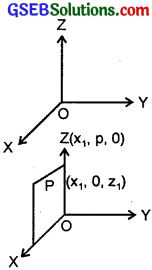# GSEB Solutions Class 11 Maths Chapter 12 Introduction to three Dimensional Geometry Ex 12.1

Gujarat Board GSEB Textbook Solutions Class 11 Maths Chapter 12 Introduction to three Dimensional Geometry Ex 12.1 Textbook Questions and Answers.

## Gujarat Board Textbook Solutions Class 11 Maths Chapter 12 Introduction to three Dimensional Geometry Ex 12.1

Question 1.
A point is on the x-axis. What are its y-coordinate and z-coordinate?
Solution:
Any point on x-axis is (x1, 0, 0), i.e., y-coordinate and z-coordinate of this point are 0 and 0 respectively.Question 2.
A point is in the xz-plane. What can you say about its y-coordinate?Solution:
Any point lying on xz-plane is (x1, 0, z1), i.e., Its y-coordinate is zero.Question 3.
Name the octant in which the following points lie (1, 2, 3), (4, – 2, 3), (4, – 2, – 5), (4, 2, – 5), (- 4, 2, – 5), (- 4, 2, 5), (- 3, – 1, 6), (2, – 4, – 7).
Solution:
The octants in which the given points lie.
(i) (1, 2, 3) : I
(ii) (4, – 2, 3) : IV
(iii) (4, – 2, – 5) : VIII
(iv) (4, 2, – 5) : V
(v) (- 4, 2, – 5) : VIX
(vi) (- 4, 2, 5) : IIX
(vii) (- 3, – 1, 6) : IIIX
(viii) (2, – 4, – 7) : VIII4. Fill in the blanks:

1. The x-axis and y-axis taken together determine a plane known as …………………..
2. Coordinates of points in XY-plane are of the form ………………………
3. Coordinate planes divide the space into ……………………… octants

Solution:

1. The x-axis and y-axis together determine a plane known as XY-plane.
2. Coordinates of points in XY-plane are of the form (x1, y1, 0).
3. Coordinate planes divides the space into 8 octants.